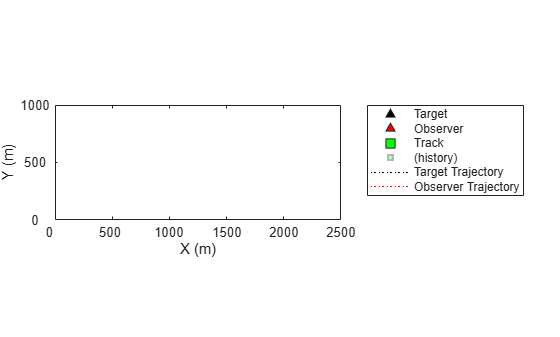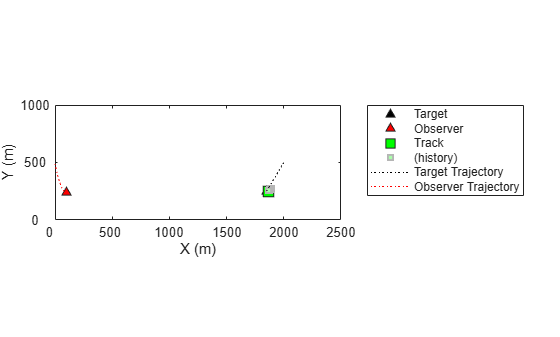# initcvmscekf

Constant velocity `trackingMSCEKF` initialization

## Syntax

``mscekf = initcvmscekf(detection)``
``mscekf = initcvmscekf(detection,rangeEstimation)``

## Description

example

````mscekf = initcvmscekf(detection)` initializes a `trackingMSCEKF` class (extended Kalman filter for tracking in modified spherical coordinates) based on information provided in an `objectDetection` object, `detection`. The function assumes a target range of 3e4 units and a range-covariance of 1e10 units2.The `trackingMSCEKF` object can be used with trackers for tracking targets with angle-only measurements from a single observer.```

example

````mscekf = initcvmscekf(detection,rangeEstimation)` allows specifying the range information to the filter. The `rangeEstimation` variable is a two-element vector, where the first element specifies the range of the target, and the second element specifies the standard deviation in range.```

## Examples

collapse all

Create an angle-only detection.

```detection = objectDetection(0,[30;20],'MeasurementParameters',... struct('Frame','Spherical','HasRange',false));```

Use `initcvmscekf` to create a `trackingMSCEKF` filter initialized using the angle-only detection.

`filter = initcvmscekf(detection)`
```filter = trackingMSCEKF with properties: State: [6x1 double] StateCovariance: [6x6 double] StateTransitionFcn: @constvelmsc StateTransitionJacobianFcn: @constvelmscjac ProcessNoise: [3x3 double] HasAdditiveProcessNoise: 0 ObserverInput: [3x1 double] MeasurementFcn: @cvmeasmsc MeasurementJacobianFcn: @cvmeasmscjac MeasurementNoise: [2x2 double] HasAdditiveMeasurementNoise: 1 ```

Create measurement parameters for subsequent rotation.

```measParamSensorToPlat = struct('Frame','Spherical','HasRange',false,... 'Orientation',rotmat(quaternion([0 0 30],'rotvecd'),'frame'))```
```measParamSensorToPlat = struct with fields: Frame: 'Spherical' HasRange: 0 Orientation: [3x3 double] ```
```measParamPlatToScenario = struct('Frame','Rectangular','HasRange',false,... 'Orientation',rotmat(quaternion([30 0 0],'rotvecd'),'frame'))```
```measParamPlatToScenario = struct with fields: Frame: 'Rectangular' HasRange: 0 Orientation: [3x3 double] ```
```measParam = [measParamSensorToPlat;measParamPlatToScenario]; detection = objectDetection(0,[30;20],'MeasurementParameters',measParam);```

Initialize a filter.

`filter = initcvmscekf(detection);`

Check that filter's measurement is same as detection.

`cvmeasmsc(filter.State,measParam)`
```ans = 2×1 30.0000 20.0000 ```

Consider a scenario when the target is moving at a constant velocity along and the observer is moving at a constant acceleration. Define target's initial state using a constant velocity model.

`tgtState = [2000;-3;500;-5;0;0];`

Define observer's initial state using a constant acceleration model.

`observerState = [0;2;0;490;-10;0.2;0;0;0];`

Create a `trackerGNN` object to use with `initcvmscekf` with some prior information about range and range-covariance.

```range = 1000; rangeStdDev = 1e3; rangeEstimate = [range rangeStdDev]; tracker = trackerGNN('FilterInitializationFcn',@(det)initcvmscekf(det,rangeEstimate));```

Simulate synthetic data by using measurement models. Get `az` and `el` information using the `cvmeas` function.

```syntheticParams = struct('Frame','Spherical','HasRange',false,... 'OriginPosition',observerState(1:3:end)); meas = cvmeas(tgtState,syntheticParams);```

Create an angle-only objectDetection to simulate synthetic detection.

```detection = objectDetection(0,meas,'MeasurementParameters',... struct('Frame','Spherical','HasRange',false),'MeasurementNoise',0.033*eye(2));```

Create trackPlotter and platformPlotter to visualize the scenario.

```tp = theaterPlot('XLimits',[0 2500],'YLimits',[0 1000]); targetPlotter = platformPlotter(tp,'DisplayName','Target','MarkerFaceColor','k'); observerPlotter = platformPlotter(tp,'DisplayName', 'Observer','MarkerFaceColor','r'); trkPlotter = trackPlotter(tp,'DisplayName','Track','MarkerFaceColor','g','HistoryDepth',50); tgtTrajPlotter = trajectoryPlotter(tp,'DisplayName','Target Trajectory','Color','k'); obsTrajPlotter = trajectoryPlotter(tp,'DisplayName','Observer Trajectory','Color','r');```Run the tracker.

```time = 0; dT = 0.1; tgtPoses = []; obsPoses = []; while time < 50 [confTracks,tentTracks,allTracks] = tracker(detection,time); for i = 1:numel(allTracks) setTrackFilterProperties(tracker,allTracks(i).TrackID,'ObserverInput',observerState(3:3:end)); end % Update synthetic detection. observerState = constacc(observerState,dT); tgtState = constvel(tgtState,dT); syntheticParams.OriginPosition = observerState(1:3:end); detection.Measurement = cvmeas(tgtState,syntheticParams); time = time + dT; detection.Time = time; % Update plots tgtPoses = [tgtPoses;tgtState(1:2:end)']; %#ok obsPoses = [obsPoses;observerState(1:3:end)']; %#ok targetPlotter.plotPlatform(tgtState(1:2:end)'); observerPlotter.plotPlatform(observerState(1:3:end)'); tgtTrajPlotter.plotTrajectory({tgtPoses}); obsTrajPlotter.plotTrajectory({obsPoses}); % Plot the first track as there are no false alarms, this should be % the target. % Get positions from the MSC state of the track. cartState = cvmeasmsc(allTracks(i).State,'rectangular') + observerState(1:3:end); trkPlotter.plotTrack(cartState'); end```## Input Arguments

collapse all

Detection report, specified as an `objectDetection` object.

Example: ```detection = objectDetection(0,[1;4.5;3],'MeasurementNoise', [1.0 0 0; 0 2.0 0; 0 0 1.5])```

Range information, specified as a two-element vector, where the first element specifies the range of the target, and the second element specifies the standard deviation in range.

Data Types: `single` | `double`

## Output Arguments

collapse all

Constant velocity tracking extended Kalman filter in an MSC frame, returned as a `trackingMSCEKF` object.

## Algorithms

• The function configures the filter with process noise assuming a unit target acceleration standard deviation.

• The function configures the covariance of the state in an MSC frame by using a linear transformation of covariance in a Cartesian frame.

• You can use this function as the `FilterInitializationFcn` property of `trackerTOMHT` and `trackerGNN` System objects.

• The function initializes the `ObserverInput` of the `trackingMSCEKF` class with zero observer acceleration in all directions. You must use the `setTrackFilterProperties` function of the trackers to update the `ObserverInput`.

## Extended Capabilities

### C/C++ Code GenerationGenerate C and C++ code using MATLAB® Coder™.

Introduced in R2018b